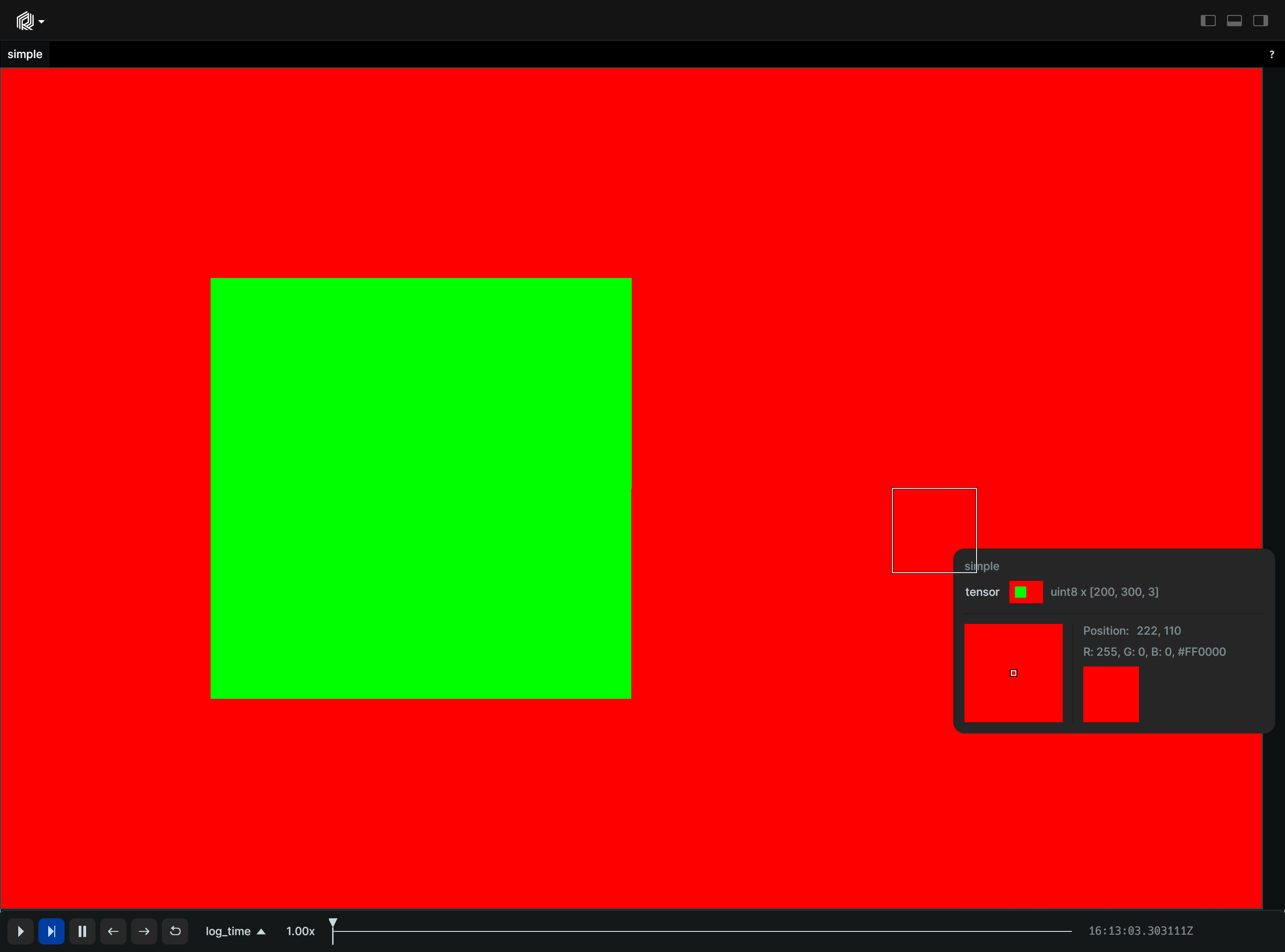# Image

A monochrome or color image.

The shape of the `TensorData` must be mappable to:

• A `HxW` tensor, treated as a grayscale image.
• A `HxWx3` tensor, treated as an RGB image.
• A `HxWx4` tensor, treated as an RGBA image.

Leading and trailing unit-dimensions are ignored, so that `1x640x480x3x1` is treated as a `640x480x3` RGB image.

## Components

Required: `TensorData`

Optional: `DrawOrder`

## Example

### image_simple

``````"""Create and log an image."""

import numpy as np
import rerun as rr

# Create an image with numpy
image = np.zeros((200, 300, 3), dtype=np.uint8)
image[:, :, 0] = 255
image[50:150, 50:150] = (0, 255, 0)

rr.init("rerun_example_image_simple", spawn=True)

rr.log("image", rr.Image(image))
``````